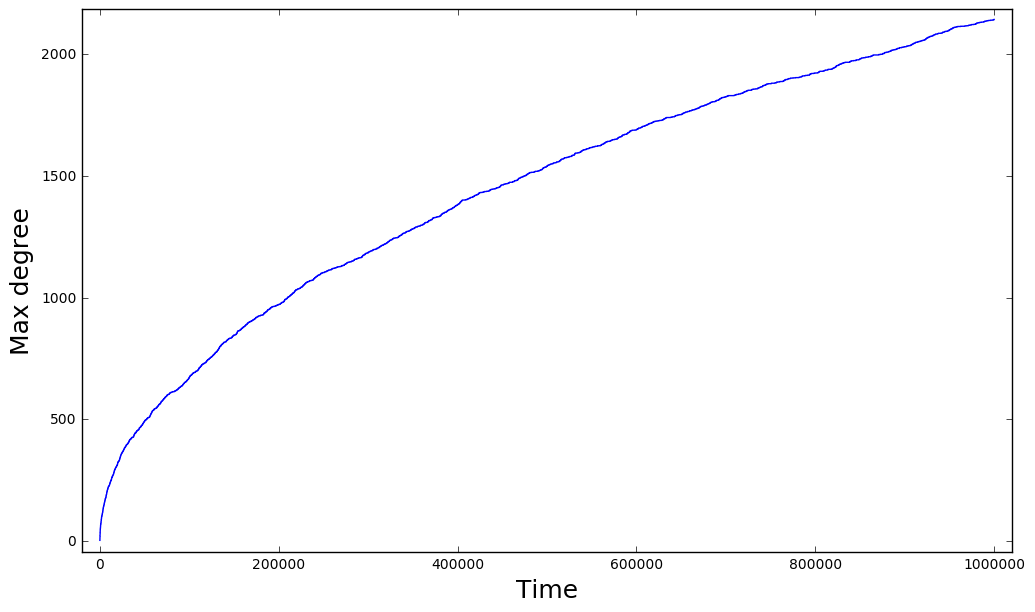In :
import numpy as np
import random as rnd
import matplotlib.pyplot as plt

%matplotlib inline

In :
#this function generates the BA scale free graph and returns how the max degree increased trough time
#parametrs: t=time, m0 initial node number (FC), m number of links for every node
#returns: max_degrees
def get_SF_maxdegree(t, m=2, m0=2):
#degree dict
degrees = {}
#max degree node in t
mdn = 0
#max degree in t
md  = m0-1
# contains the nodes as many times as it's degree
nodeList = []
# max degrees
max_degrees = []
#initialize the FC graph at t=0
for i in range(m0):
# connecting the node with all of the other nodes
#edges[i] = set([j for j in range(m0) if i!=j])
# adding the i node to nodeList as many times as it's degree
nodeList.extend([i]*m0)
degrees[i] = m0
#iterating trough time, time step also used as node index
#but I already have 0,..., m0 nodes, so I shift the loop
for i in range(m0,t+m0):
#selected nodes: this nodes will be connected to the new node
selected_nodes = []
#we try to select node until we have selected m node
while len(selected_nodes)!=m:
# selecting a node, nodeList contains a node as many times as it's degree.
# So the probability of selecting node is propto it's degree.
sn = nodeList[rnd.randint(0, len(nodeList)-1)]
# if we already selected this node, we don't select it again,
# because we can't connect multiple times the same two node
if sn not in selected_nodes:
# adding the selected node to the selected_nodes list
selected_nodes.append(sn)
# if sn is the max degree node, then de max degree is increased now with 1
if sn==mdn:
md += 1
degrees[sn] += 1
# if the new node degree is bigger then md then we have a new max degree node
if degrees[sn]>md:
md  = degrees[sn]
mdn = sn
# adding the selected nodes to nodeList
nodeList.extend(selected_nodes)
# adding the new node to the nodeList as many times as it's degree, which is m
nodeList.extend([i]*m)
# adding the new node to degrees dict
degrees[i] = m
# if the new node degree's > max degree: we have a new max degree node
if m>md:
md  = m
mdn = i
max_degrees.append(md)
return max_degrees

In :
# getting the degree increase for t=10^6
degrees = get_SF_maxdegree(1000000)

In :
# creating the time list
time = [i for i in range(len(degrees))]

In :
#plot
plt.figure(0, (12,7))
plt.xlim([-max(time)*0.02, max(time)*1.02])
plt.ylim([-max(degrees)*0.02, max(degrees)*1.02])
plt.xlabel("Time", fontsize=18)
plt.ylabel("Max degree", fontsize=18)
plt.plot(time, degrees)

Out:
[<matplotlib.lines.Line2D at 0x1f71c05e4a8>]